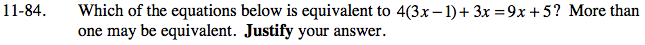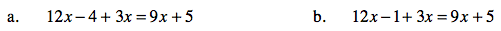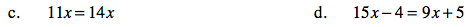Home > AC > Chapter 11 > Lesson 11.2.1 > Problem11-84

11-84.
1. Which of the equations below is equivalent to 4(3x − 1) + 3x = 9x + 5? More than one may be equivalent. Justify your answer. Homework Help ✎

1. 12x − 4 + 3x = 9x + 5

2. 12x − 1 + 3x = 9x + 5

3. 11x = 14x

4. 15x − 4 = 9x + 5Write out the steps for solving the original equation. Which of the equations match one of your steps?